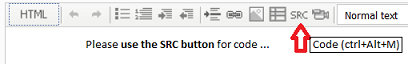Indicator help needed106

extern datetime candledate=D'05.07.2016 16:00';//DD.MM.YYYY. HH:MM

//---- buffer
double pips;
//+------------------------------------------------------------------+
//|                                                                  |
//+------------------------------------------------------------------+
int OnInit()
{
pips=Point; //.00001 or .001
if(Digits==3 || Digits==5)
pips*=10; // pips=0,0001 or pips=0,01
//+------------------------------------------------------------------+
SetIndexStyle(0,DRAW_LINE,STYLE_DASHDOTDOT,1,clrGreen);
return(INIT_SUCCEEDED);
}
//----
int OnCalculate (const int rates_total,
const int prev_calculated,
const datetime& time[],
const double& open[],
const double& high[],
const double& low[],
const double& close[],
const long& tick_volume[],
const long& volume[],

{
int limit;
if(!prev_calculated)limit=rates_total-2;
else limit=rates_total-prev_calculated;
//+------------------------------------------------------------------+

//+------------------------------------------------------------------+
int mycandle=0;
double candleclose=0.0;
//+------------------------------------------------------------------+
mycandle=iBarShift(NULL,PERIOD_M5,candledate,true);
//+------------------------------------------------------------------+
for(int i=0;i<=mycandle;i++)
{
candleclose=iClose(NULL,0,mycandle);
if(iLow(NULL,0,i)<candleclose-20*pips)
else
};

return(rates_total);
}

Hi,

I have a simply indicator which should do the following:

1. Look at an specific date for the candle
2. check the close of that candle
3. if the current low is 20 pips below the close of that candle, draw a line,
otherwise draw the line at the level of the candle's close.

Here's my problem:

Check AUDUSD M5 for the date 05.07.2016 16:00
first, the line is at the candle's close level, then the line moves to 20 pips below the level.

But later on the price moves back and also the line moves back. But I don't want the line moves back. In other words: I want the line is moving only one time - if the price goes 20 pips away. Then the line should stay at this level, it doesn't matter where the price is going after the 1st movement.

Dan

Files:26828

hilfx: if the price goes 20 pips away. Then the line should stay at this level, it doesn't matter where the price is going after the 1st movement.
1.Play video Please edit your post. For large amounts of code, attach it.

2. mycandle=iBarShift(NULL,PERIOD_M5,candledate,true);
candleclose=iClose(NULL,0,mycandle);
You are mixing apples and oranges
3. iClose(NULL,0,mycandle);
iLow(NULL,0,i)
Why use a function call when you can use the Predefined Variables - MQL4 Reference
4. Count down and don't move it back.
candleclose=iClose(NULL,0,mycandle);
for(int i=mycandle; i >= 0; --i){
if(Low[i] < candleclose-20*pips)
candleclose -= 20*pips;
//      else
};106

#property indicator_chart_window
#property indicator_buffers 1
#property strict
//----
extern datetime candledate=D'07.07.2016 07:30';//YYYY.MM.DD. HH:MM

//---- buffer
double pips;
//+------------------------------------------------------------------+
//|                                                                  |
//+------------------------------------------------------------------+
int OnInit()
{
pips=Point; //.00001 or .001
if(Digits==3 || Digits==5)
pips*=10; // pips=0,0001 or pips=0,01
//+------------------------------------------------------------------+
SetIndexStyle(0,DRAW_LINE,STYLE_DASHDOTDOT,1,clrGreen);
return(INIT_SUCCEEDED);
}
//----
int OnCalculate(const int rates_total,
const int prev_calculated,
const datetime &time[],
const double &open[],
const double &high[],
const double &low[],
const double &close[],
const long &tick_volume[],
const long &volume[],

{
int limit;
if(!prev_calculated)limit=rates_total-2;
else limit=rates_total-prev_calculated;
//+------------------------------------------------------------------+
int mycandle=iBarShift(NULL,0,candledate,true);
double candleclose=Close[mycandle];
//+------------------------------------------------------------------+
Comment("i der Kerze: ",IntegerToString(mycandle,0)
,"\ncandledate: ",TimeToString(candledate,TIME_DATE|TIME_MINUTES)
,"\nClose: ",DoubleToString(candleclose,_Digits)
,"\nTrigger: ",DoubleToString(candleclose-15*pips,_Digits)
);
//+------------------------------------------------------------------+
for(int i=mycandle;i>=0;i--)
{
if(Low[i]<=candleclose-15*pips)
candleclose-=15*pips;